International
Tables for
Crystallography
Volume E
Subperiodic groups
Edited by V. Kopský and D. B. Litvin

International Tables for Crystallography (2006). Vol. E, ch. 1.2, p. 15   | 1 | 2 |

## Section 1.2.9. Symmetry operations

V. Kopskýa and D. B. Litvinb*

aDepartment of Physics, University of the South Pacific, Suva, Fiji, and Institute of Physics, The Academy of Sciences of the Czech Republic, Na Slovance 2, PO Box 24, 180 40 Prague 8, Czech Republic, and bDepartment of Physics, Penn State Berks Campus, The Pennsylvania State University, PO Box 7009, Reading, PA 19610-6009, USA
Correspondence e-mail:  u3c@psu.edu

### 1.2.9. Symmetry operations

| top | pdf |

The coordinate triplets of the General position of a subperiodic group may be interpreted as a shorthand description of the symmetry operations in matrix notation as in the case of space groups [see Sections 2.2.3, 8.1.5and 11.1.1of IT A (2005)]. The geometric description of the symmetry operations is found in the subperiodic group tables under the heading Symmetry operations. These data form a link between the subperiodic group diagrams (Section 1.2.6) and the general position (Section 1.2.11).

#### 1.2.9.1. Numbering scheme

| top | pdf |

The numbering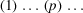of the entries in the blocks Symmetry operations and General position (first block below Positions) is the same. Each listed coordinate triplet of the general position is preceded by a number between parentheses (p). The same number (p) precedes the corresponding symmetry operation. For all subperiodic groups with primitive lattices, the two lists contain the same number of entries.

For the nine layer groups with centred lattices, to the one block of General positions correspond two blocks of Symmetry operations. The numbering scheme is applied to both blocks. The two blocks correspond to the two centring translations below the subheading Coordinates, i.e.. For the Positions, the reader is expected to add these two centring translations to each printed coordinate triplet in order to obtain the complete general position. For the Symmetry operations, the corresponding data are listed explicitly with the two blocks having the subheadings For (0, 0, 0)+ set' and For (1/2, 1/2, 0)+ set', respectively.

#### 1.2.9.2. Designation of symmetry operations

| top | pdf |

The designation of symmetry operations for the subperiodic groups is the same as for the space groups. An entry in the block Symmetry operations is characterized as follows:

 (i) A symbol denoting the type of the symmetry operation [cf. Chapter 1.2of IT A (2005)], including its glide or screw part, if present. In most cases, the glide or screw part is given explicitly by fractional coordinates between parentheses. The sense of a rotation is indicated by the superscript + or −. Abbreviated notations are used for the glide reflections a(1/2, 0, 0) ≡ a; b(0, 1/2, 0) ≡ b; c(0, 0, 1/2) ≡ c. Glide reflections with complicated and unconventional glide parts are designated by the letter g, followed by the glide part between parentheses. (ii) A coordinate triplet indicating the location and orientation of the symmetry element which corresponds to the symmetry operation. For rotoinversions the location of the inversion point is also given.

Details of this symbolism are given in Section 11.1.2of IT A (2005).

#### Examples

 (1)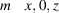: a reflection through the plane, i.e. the plane parallel to (010) containing the point (0, 0, 0). (2)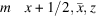: a reflection through the plane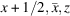, i.e. the plane parallel to (110) containing the point (1/2, 0, 0). (3)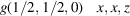: glide reflection with glide component (1/2, 1/2, 0) through the plane, i.e. the plane parallel to () containing the point (0, 0, 0). (4): screw rotation along the (100) direction containing the point (0, 1/4, 0) with a screw component (1/2, 0, 0). (5)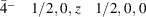: fourfold rotoinversion consisting of a clockwise rotation by 90° around the line 1/2, 0, z followed by an inversion through the point (1/2, 0, 0).

### References

International Tables for Crystallography (2005). Vol. A. Space-group symmetry, edited by Th. Hahn. Heidelberg: Springer. [Previous editions: 1983, 1987, 1992, 1995 and 2002. Abbreviated as IT A (2005).]Google Scholar